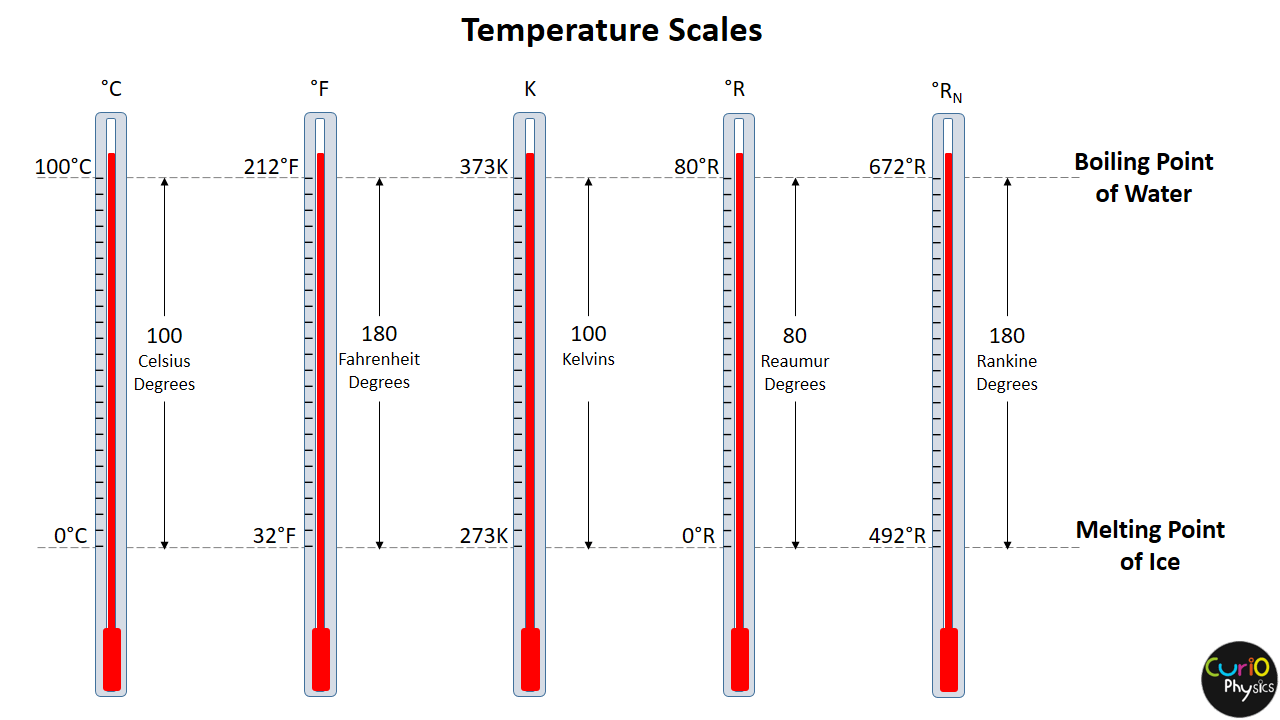# Measurement of Temperature

Measurement of Temperature :- In the previous article Temperature we discussed that for temperature measurement of an object, we need to construct a temperature scale. In that temperature scale we utilize any measurable property of a system that varies linearly over a wide range with temperature.

That measurable property for temperature measurement may be :-

• the length of mercury column (L) filled in the thermometer
• The pressure (p) of a quantity of gas in a constant volume container
• the electrical resistance R of a conductor wire

etc.

##### Measurement of Temperature with linear length variation of a rod

(1) Suppose that the length of a rod varies linearly with temperature :-At temperature T1 the length of the rod is l1 and at temperature T2 the length of the rod becomes l2. Now, if in this temperature range (T2-T1), the variation of length of the rod ( l2 to l1 ) is linear then :-$\displaystyle dl\propto dT$$\displaystyle \Rightarrow \frac{dl}{dT}=cons.$

i.e. rate of change of length with temperature = constant$\displaystyle \Rightarrow \frac{{{T}_{2}}-{{T}_{1}}}{{{l}_{2}}-{{l}_{1}}}=\frac{T-{{T}_{1}}}{l-{{l}_{1}}}$

using above relation, knowing the length of the rod (l), the corresponding temperature (T) can be calculated.

Note :- Here we are saying that dl ∝ dT ,  we cannot say l ∝ T , as then l = k T and at T = 0ºC , l = 0 m, which is wrong.

Example.

At T = 0ºC, the length of a rod is 5cm & at T = 100ºC, length of rod is 25cm. Find at what “T”, length of rod will be 10cm.

Sol.

Using$\displaystyle \frac{{{T}_{2}}-{{T}_{1}}}{{{l}_{2}}-{{l}_{1}}}=\frac{T-{{T}_{1}}}{l-{{l}_{1}}}$

we get$\displaystyle \frac{100-0}{25-5}=\frac{T-0}{10-5}$$\displaystyle \frac{100}{20}=\frac{T}{5}$

⇒ T = 25ºC

##### Measurement of Temperature with linear pressure variation of a gas

(2) If pressure of gas varies linearly with temperature then it can also be used for temperature measurement.$\displaystyle dp\propto dT$$\displaystyle \Rightarrow \frac{dp}{dT}=cons.$

i.e. rate of change of pressure with temperature = constant$\displaystyle \Rightarrow \frac{{{T}_{2}}-{{T}_{1}}}{{{p}_{2}}-{{p}_{1}}}=\frac{T-{{T}_{1}}}{p-{{p}_{1}}}$

knowing the pressure (p), the corresponding temperature (T) can be calculated.

##### Measurement of Temperature with Linear Volume Variation of a gas

(3) Using Linear Volume Variation of a gas for temperature measurement,$\displaystyle dV\propto dT$$\displaystyle \Rightarrow \frac{dV}{dT}=cons.$

i.e. rate of change of volume with temperature = constant$\displaystyle \Rightarrow \frac{{{T}_{2}}-{{T}_{1}}}{{{V}_{2}}-{{V}_{1}}}=\frac{T-{{T}_{1}}}{V-{{V}_{1}}}$

knowing the volume (V), the corresponding temperature (T) can be calculated.

##### Measurement of Temperature with linear resistance variation of a wire

(4) If the resistance of a wire varies directly with the changes in temperature, then resistance can also be used for temperature measurement.$\displaystyle dR\propto dT$$\displaystyle \Rightarrow \frac{dR}{dT}=cons.$

i.e. rate of change of resistance with temperature = constant$\displaystyle \Rightarrow \frac{{{T}_{2}}-{{T}_{1}}}{{{R}_{2}}-{{R}_{1}}}=\frac{T-{{T}_{1}}}{R-{{R}_{1}}}$

knowing the resistance (R) of the wire, the corresponding temperature (T) can be calculated from above relation.